# LightCAKE: A Lightweight Framework for Context-Aware Knowledge Graph Embedding

2021.03.09: 修正关于引入逆三元组的影响.

2021.04.18: 更新一篇更早的类似论文GAKE.

# LightCAKE: A Lightweight Framework for Context-Aware Knowledge Graph Embedding

## Basic Idea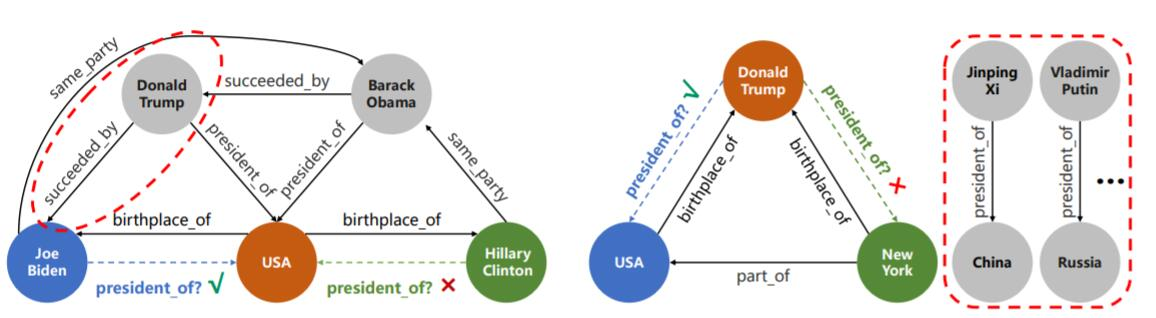## LightCAKE

### Context Star Graph

• Entity Context: 对于头实体$h$, 实体上下文被定义为$h$ 的邻居, $\mathcal{C}_{\text {ent }}(h)=\{(r, t) \mid(h, r, t) \in \mathcal{G}\}$.
• Relation Context: 对于关系$r$, 关系上下文被定义为$r$ 下的全部头尾实体对, $\mathcal{C}_{r e l}(r)=\{(h, t) \mid(h, r, t) \in \mathcal{G}\}$.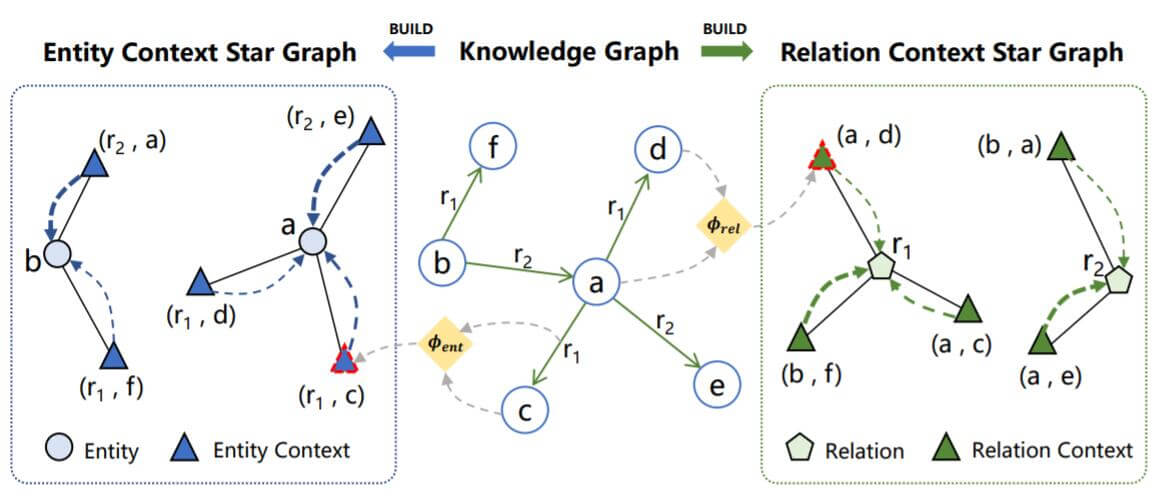Inverse Triplet是否会导致Inverse Leakage?

### LightCAKE Details

LightCAKE被作者分为两部分:

1. 将实体或关系上下文编码进Embedding(Encode).
2. 迭代聚合上下文节点的信息(Attention).

$$\begin{array}{l} e_{h}^{(l+1)}=e_{h}^{(l)}+\sum\limits_{\left(r^{\prime}, t^{\prime}\right) \in \mathcal{C}_{\text {ent }}(h)} \alpha_{h,\left(r^{\prime}, t^{\prime}\right)}^{(l)} \phi_{\text {ent }}\left(e_{r^{\prime}}, e_{t^{\prime}}\right) \\ e_{r}^{(l+1)}=e_{r}^{(l)}+\sum\limits_{\left(h^{\prime}, t^{\prime}\right) \in \mathcal{C}_{r e l}(r)} \beta_{r,\left(h^{\prime}, t^{\prime}\right)}^{(l)} \phi_{\text {rel }}\left(e_{h^{\prime}}, e_{t^{\prime}}\right) \end{array}$$

$\alpha_{h,\left(r^{\prime}, t^{\prime}\right)}^{(l)}, \beta_{r,\left(h^{\prime}, t^{\prime}\right)}^{(l)}$ 分别是在实体上下文星图和关系上下文星图中对其他节点的注意力:
\begin{aligned} \alpha_{h,\left(r^{\prime}, t^{\prime}\right)}^{(l)}=\frac{\exp \left(\psi\left(e_{h}^{(l)}, e_{r^{\prime}}^{(l)}, e_{t^{\prime}}^{(l)}\right)\right)}{\sum_{\left(r^{\prime \prime}, t^{\prime \prime}\right) \in \mathcal{C}_{e n t}(h)} \exp \left(\psi\left(e_{h}^{(l)}, e_{r^{\prime \prime}}^{(l)}, e_{t^{\prime \prime}}^{(l)}\right)\right)} \\ \beta_{r,\left(h^{\prime}, t^{\prime}\right)}^{(l)}=\frac{\exp \left(\psi\left(e_{h^{\prime}}^{(l)}, e_{r}^{(l)}, e_{t^{\prime}}^{(l)}\right)\right)}{\sum_{\left(h^{\prime \prime}, t^{\prime \prime}\right) \in \mathcal{C}_{r e l}(r)} \exp \left(\psi\left(e_{h^{\prime \prime}}^{(l)}, e_{r}^{(l)}, e_{t^{\prime \prime}}^{(l)}\right)\right)} \end{aligned}

$$p(r \mid h, t)=\frac{\exp \left(\psi\left(e_{h}^{(L)}, e_{r}^{(L)}, e_{t}^{(L)}\right)\right)}{\sum_{r^{\prime} \in \mathcal{R}} \exp \left(\psi\left(e_{h}^{(L)}, e_{r^{\prime}}^{(L)}, e_{t}^{(L)}\right)\right)}$$

$$\mathcal{L}=-\frac{1}{|\mathcal{D}|} \sum_{i=0}^{|\mathcal{D}|} \log p\left(r_{i} \mid h_{i}, t_{i}\right)$$

## Experiments

### Main Results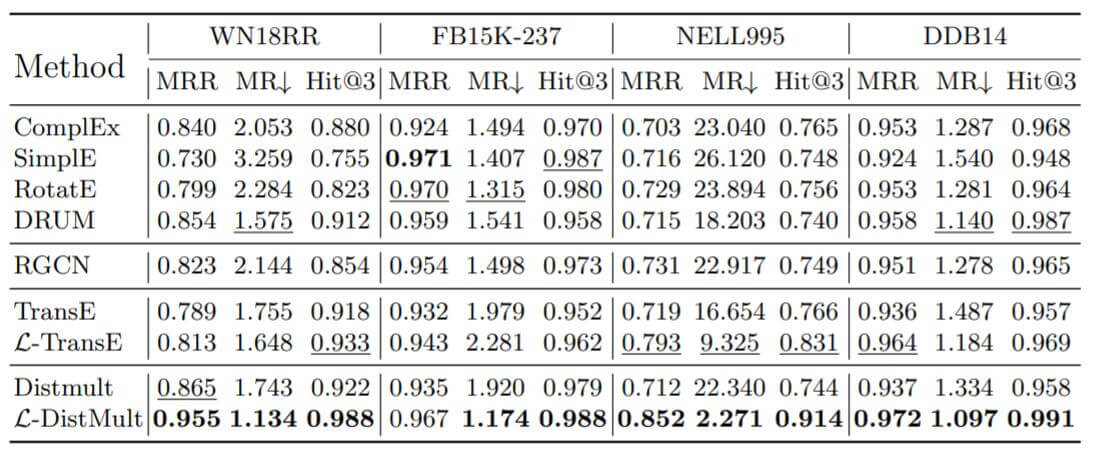### Ablation Study and Analysis on Number of Iterations

1. 不引入任何图结构的信息.
2. 只引入关系上下文信息, 记为$\mathcal{L}_{rel}$.
3. 只引入实体上下文信息, 记为$\mathcal{L}_{ent}$.
4. 引入实体和关系上下文信息, 记为$\mathcal{L}$.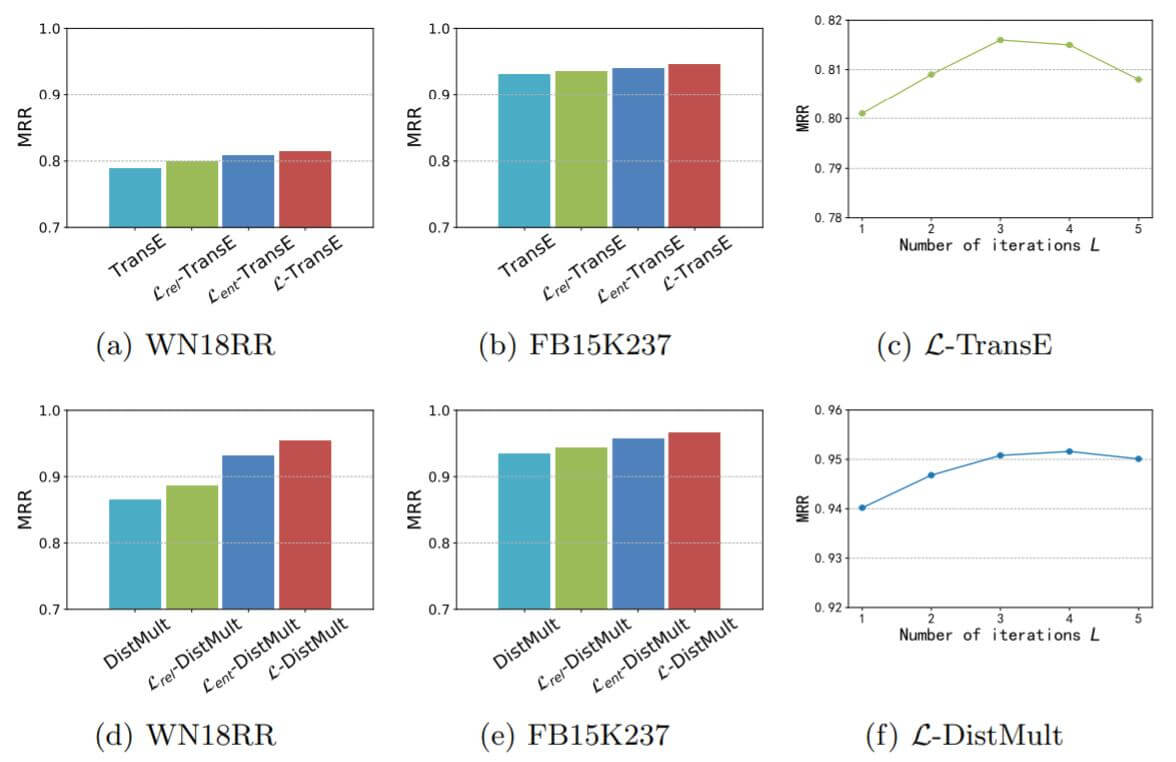### Efficiency Analysis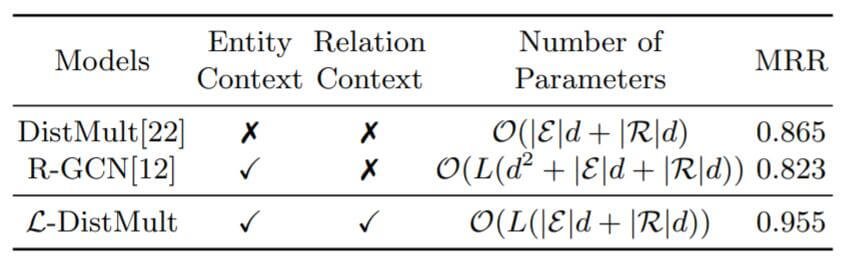DistMult在改进后增加了L倍的复杂度, 但却获得了实体和关系上的图结构信息双加成, 效果提升显著. 而R - GCN的时间复杂度比较高, 没有利用图上的关系信息.

## Summary

LightCAKE是一个轻量级集成图多跳信息的KGE框架, 无需添加任何额外的参数, 仅用部分计算量就将关系和实体上下文信息全部集成了起来, 整体非常简洁. 作者也注意到了GNN中的过参数化问题, 这点很宝贵. 但实验部分采用了Relation Prediction作为评估, 我仍然期待它在Link Prediction上的效果.

1. 要利用好图结构优势, 图中蕴含着多种多跳信息, 不能白白浪费它们. 从可解释的角度, 图结构也占有优势.
2. 注意各类方法在KGE中的过参数化(尤其是GNN), 有时候参数过多也不是啥好事, 可能不利于优化, 还增加了时间复杂度.
3. 引入实体, 关系双角度信息, 能更好的刻画节点. 如果有更多角度的信息, 能更精确的找到节点定位.

上一篇ConvR: Adaptive Convolution for Multi-Relational Learning

2021-03-09Introduction: Graph Neural Network

2021-03-04
目录